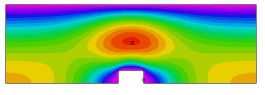﻿ Sample Problems > Applications > Fluids > viscous

# viscous

Navigation:  Sample Problems > Applications > Fluids >

# viscous{  VISCOUS.PDE

This example shows the application of FlexPDE to problems in

viscous flow.

The Navier-Stokes equation for steady incompressible flow in two

cartesian dimensions is

dens*(dt(U) + U*dx(U) + V*dy(U)) = visc*del2(U) - dx(P) + dens*Fx

dens*(dt(V) + U*dx(V) + V*dy(V)) = visc*del2(V) - dy(P) + dens*Fy

together with the continuity equation

div[U,V] = 0

where

U and V are the X- and Y- components of the flow velocity

P is the fluid pressure

dens is the fluid density

visc is the fluid viscosity

Fx and Fy are the X- and Y- components of the body force.

In principle, the third equation enforces incompressible mass conservation,

but the equation contains no reference to the pressure, which is nominally

the remaining variable to be determined.

In practice, we choose to solve a "slightly compressible" system by defining a

hypothetical equation of state

p(dens) = p0 + L*(dens-dens0)

where p0 and dens0 represent a reference density and pressure, and L is a large

number representing a strong response of pressure to changes of density.  L is

chosen large enough to enforce the near-incompressibility of the fluid, yet not

so large as to erase the other terms of the equation in the finite precision

of the computer arithmetic.

The compressible form of the continuity equation is

dt(dens) + div(dens*U) = 0

which, together with the equation of state yields

dt(p) = -L*dens0*div(U)

[see Help | Tech Notes | Smoothing Operators in PDEs"],

resulting in the final pressure equation:

Here M has the dimensions of density/time or viscosity/distance^2.

The problem posed here shows flow in a 2D channel blocked by a bar of square

cross-section.  The channel is mirrored on the bottom face, and only the upper

half is computed.

We have chosen a "convenient" value of M, one that gives good

accuracy in reasonable time.  The user can alter this value to find one

which is satisfactory for his application.

We have included three elevation plots of X-velocity, at the inlet, center

and outlet of the channel.  The integrals presented on these plots show the

consistency of mass transport across the channel.

}

title 'Viscous flow in 2D channel, Re < 0.1'

 variables   u(0.1)   v(0.01)   p(1)   select   ngrid = 40   definitions   Lx = 5       Ly = 1.5   Gx = 0       Gy = 0   p0 = 1   speed2 = u^2+v^2   speed = sqrt(speed2)   dens = 1   visc = 1vxx = (p0/(2*visc*Lx))*(Ly-y)^2 { open-channel x-velocity }

rball=0.25

cut = 0.05   { value for bevel at the corners of the obstruction }

pfactor = staged(1,10,100,1000)

penalty = pfactor*visc/rball^2   { "equation of state" }

Re = globalmax(speed)*(Ly/2)/(visc/dens)

initial values

u = 0.5*vxx  v = 0  p = p0*x/Lx

equations

u:  visc*div(grad(u)) - dx(p) = dens*(u*dx(u) + v*dy(u))

v:  visc*div(grad(v)) - dy(p) = dens*(u*dx(v) + v*dy(v))

boundaries

region 1

start(0,0)

{ unspecified boundary conditions default to LOAD=0 }

value(v)=0   line to (Lx/2-rball,0)

value(u)=0   line to (Lx/2-rball,rball) bevel(cut)

line to (Lx/2+rball,rball) bevel(cut)

line to (Lx/2+rball,0)

value(p)=p0 line to (Lx,Ly)

monitors

contour(speed) report(Re)

plots

grid(x,y)

contour(u) report(Re)

contour(v) report(Re)

contour(speed) painted report(Re)

vector(u,v) as "flow"   report(Re)

contour(p) as "Pressure" painted

contour(dx(u)+dy(v)) as "Continuity Error"

contour(p) zoom(Lx/2,0,1,1) as "Pressure"

elevation(u) from (0,0) to (0,Ly)

elevation(u) from (Lx/2,0) to (Lx/2,Ly)

elevation(u) from (Lx,0) to (Lx,Ly)

end